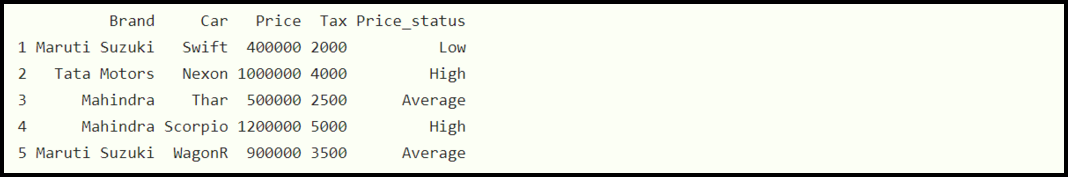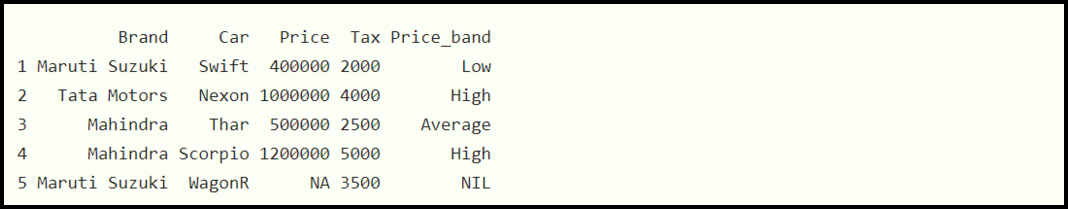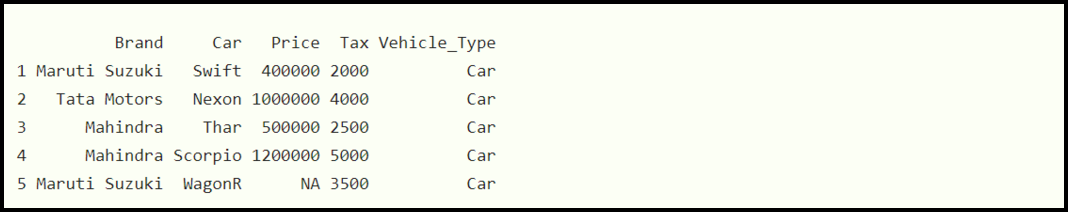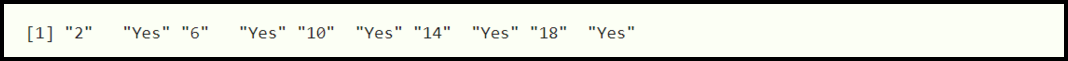GeeksforGeeks App
Open AppBrowser
Continue

# Case when statement in R Dplyr Package using case_when() Function

This article focuses upon the case when statement in the R programming language using the case_when() function from the Dplyr package.

Case when is a mechanism using which we can vectorize a bunch of if and else if statements. In simple words, using a case when statement we evaluate a condition expression, and based on that we make decisions. For example, suppose we want to check whether a candidate is eligible to cast a vote.  To solve this problem, we can evaluate his age and if it is greater than 18 we will allow him to vote otherwise he is not eligible.

## Case when in R:

R provides us case_when() function using which we can implement case when in R. It is equivalent to “case when” statement in SQL.

Syntax:

case_when(expression)

Here,

• expression: It represent a condition expression

## Method 1: Create a new variable after executing  the case when statement and using mutate function:

Mutate function in R is used to add newly created variables and it also preserves the existing variables.

Example:

In this example, we are creating a data frame that holds car brands, names, prices, taxes. Now with the help of mutate() function, we are creating an additional column (Price_Status) that will store string literals: high, average, and low after evaluating the price used inside the case_when() function.

## R

 `# Creating a new variable using case_when() and mutate() function`` ` `# Import the library``library``(dplyr)`` ` `# Creating a dataframe``data_frame = ``data.frame``(Brand=``c``(``"Maruti Suzuki"``, ``"Tata Motors"``,``                                ``"Mahindra"``, ``"Mahindra"``, ``"Maruti Suzuki"``),``                        ``Car=``c``(``"Swift"``, ``"Nexon"``, ``"Thar"``, ``"Scorpio"``, ``"WagonR"``),``                        ``Price=``c``(400000, 1000000, 500000, 1200000, 900000),``                        ``Tax=``c``(2000, 4000, 2500, 5000, 3500))`` ` `# Using case_when() to create new variable``data_frame % > % ``mutate``(Price_status=``case_when``(Price >= 500000 & Price <= 900000 ~ ``"Average"``, Price > 900000 ~ ``"High"``, ``TRUE` `~ ``"Low"``))`

Output:## Method 2: Handling NA using Case when statement

Look into the Price column of the data_frame that we have created above once again. Some cars have a price value equal to NA. While applying case_when() function, this must be handled carefully. R provides us is.na() function using which we can handle na values.

Example:

In this example, we are creating a data frame that holds car brands, names, prices, taxes. Now with the help of mutate() function, we are creating an additional column (Price_Status) that will store string literals: high, average, and low after evaluating the price used inside the case_when() function. Note that for cars having the price equal to NA we are adding “NIL”, at the corresponding position of the Price_Status column.

## R

 `# Creating a new variable using case_when() and mutate() function``# and handling unwanted values`` ` `# Import the library``library``(dplyr)`` ` `data_frame = ``data.frame``(Brand=``c``(``"Maruti Suzuki"``, ``"Tata Motors"``,``                                ``"Mahindra"``, ``"Mahindra"``, ``"Maruti Suzuki"``),``                        ``Car=``c``(``"Swift"``, ``"Nexon"``, ``"Thar"``, ``"Scorpio"``, ``"WagonR"``),``                        ``Price=``c``(400000, 1000000, 500000, 1200000, ``NA``),``                        ``Tax=``c``(2000, 4000, 2500, 5000, 3500))`` ` ` ` `# Case_when() to create new variable (or column) with NIL``data_frame % > % ``mutate``(Price_band=``case_when``(``is.na``(Price) ~ ``"NIL"``, Price >= 500000 & Price <= 900000   ~ ``"Average"``, Price > 900000 ~ ``"High"``, ``TRUE` `~ ``"Low"``))`

Output:## Method 3: Using switch statement in R

R allows us to use sapply() with a switch statement to construct a new variable that can exist as a column in the data frame.

Example:

In this example, we are We have created an additional column with the name “Vehicle_Type” we are using sapply() function with a switch statement and for respective Brands, we are marking the values of the at the corresponding position of the Vehicle_Type column as “Car”.

## R

 `# R program using case_when through sapply() function`` ` `# Import the library``library``(dplyr)`` ` `# Creating a dataframe``data_frame = ``data.frame``(Brand = ``c``(``"Maruti Suzuki"``,``"Tata Motors"``,``                                  ``"Mahindra"``,``"Mahindra"``, ``"Maruti Suzuki"``),``Car = ``c``(``"Swift"``,``"Nexon"``,``"Thar"``, ``"Scorpio"``, ``"WagonR"``),``Price = ``c``(400000,1000000,500000,1200000,``NA``),``Tax = ``c``(2000,4000,2500,5000,3500))`` ` `# Case_when() to create new variable``data_frame\$Vehicle_Type <- ``sapply``(data_frame\$Brand, switch, ``"Tata Motors"``=``'Car'``, ``                                  ``"Mahindra"``=``'Car'``, ``"Maruti Suzuki"` `= ``'Car'``)`` ` `data_frame`

Output:## Method 4: Using case_when in vector

R also provides the facility to use case_when for manipulating a vector.

Example:

Consider the below source code. In this example, we are first checking whether the current value in the vector is divisible by 4, and if it is so then we are he replacing the multiples of 4 with the string “Yes”.

Example:

## R

 `# R program using case_when() function to manipulate a vector`` ` `# Importing library``library``(dplyr)`` ` `# Creating a vector``vector <- ``seq``(2, 20, by = 2)`` ` `# Using case_when() function``case_when``(``   ` `  ``# If the value is divisible by 4 ``  ``# then replace it with "Yes"``  ``vector %% 4 == 0 ~ ``"Yes"``,``  ``TRUE` `~ ``as.character``(vector)``)`

Output:Using case_when in vector

My Personal Notes arrow_drop_up